# Area

﻿
Area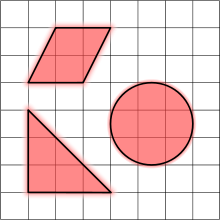The combined area of these three shapes is between 15 and 16 squares.

Area is a quantity that expresses the extent of a two-dimensional surface or shape in the plane. Area can be understood as the amount of material with a given thickness that would be necessary to fashion a model of the shape, or the amount of paint necessary to cover the surface with a single coat. It is the two-dimensional analog of the length of a curve (a one-dimensional concept) or the volume of a solid (a three-dimensional concept).

The area of a shape can be measured by comparing the shape to squares of a fixed size. In the International System of Units (SI), the standard unit of area is the square metre (m2), which is the area of a square whose sides are one metre long. A shape with an area of three square metres would have the same area as three such squares. In mathematics, the unit square is defined to have area one, and the area of any other shape or surface is a dimensionless real number.

There are several well-known formulas for the areas of simple shapes such as triangles, rectangles, and circles. Using these formulas, the area of any polygon can be found by dividing the polygon into triangles. For shapes with curved boundary, calculus is usually required to compute the area. Indeed, the problem of determining the area of plane figures was a major motivation for the historical development of calculus.

For a solid shape such as a sphere, cone, or cylinder, the area of its boundary surface is called the surface area. Formulas for the surface areas of simple shapes were computed by the ancient Greeks, but computing the surface area of a more complicated shape usually requires multivariable calculus.

Area plays a important role in modern mathematics. In addition to its obvious importance in geometry and calculus, area is related to the definition of determinants in linear algebra, and is a basic property of surfaces in differential geometry. In analysis, the area of a subset of the plane is defined using Lebesgue measure, though not every subset is measurable. In general, area in higher mathematics is seen as a special case of volume for two-dimensional regions.

## Formal definition

An approach to defining what is meant by area is through axioms. For example, we may define area as a function a from a collection M of special kind of plane figures (termed measurable sets) to the set of real numbers which satisfies the following properties:

• For all S in M,$a(S) \ge 0$.
• If S and T are in M then so are$S\cup T$ and$S\cap T$ and also,$a(S\cup T)=a(S)+a(T)-a(S\cap T)$.
• If S and T are in M with$S\subset T$ then TS is in M and a(TS) = a(T) − a(S).
• If a set S is in M and S is congruent to T then T is also in M and a(S) = a(T).
• Every rectangle R is in M. If the rectangle has length h and breadth k then a(R) = hk.
• Let Q be a set enclosed between two step regions S and T. A step region is formed from a finite union of adjacent rectangles resting on a common base, i.e.$S\subset Q\subset T$. If there is a unique number c such that$a(S)\le c\le a(T)$ for all such step regions S and T, then a(Q) = c.

It can be proved that such a area function actually exists. (See, for example, Elementary Geometry from an Advanced Standpoint by Edwin Moise.)

## Units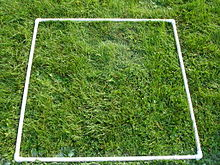Every unit of length has a corresponding unit of area, namely the area of a square with the given side length. Thus areas can be measure in square metres (m2), square centimetres (cm2), square millimetres (mm2), square kilometres (km2), square feet (ft2), square yards (yd2), square miles (mi2), and so forth. Algebraically, these units can be thought of as the squares of the corresponding length units.

The SI unit of area is the square metre, which is considered an SI derived unit.

### ConversionsAlthough there are 10 mm in 1 cm, there are 100 mm2 in 1 cm2.

The conversion between two square units is the square of the conversion between the corresponding length units. For example, since

1 foot = 12 inches,

the relationship between square feet and square inches is

1 square foot = 144 square inches,

where 144 = 122 = 12 × 12. Similarly:

• 1 square kilometer = 1,000,000 square meters
• 1 square meter = 10,000 square centimetres = 1,000,000 square millimetres
• 1 square centimetre = 100 square millimetres
• 1 square yard = 9 square feet
• 1 square mile = 3,097,600 square yards = 27,878,400 square feet

• 1 square inch = 6.4516 square centimetres
• 1 square foot = 0.09290304 square metres
• 1 square yard = 0.83612736 square metres
• 1 square mile = 2.589988110336 square kilometres

### Other units

There are several other common units for area. The are was the original unit of area in the metric system, with

• 1 are = 100 square metres

Though the are has fallen out of use, the hectare is still commonly used to measure land:

• 1 hectare = 100 ares = 10,000 square metres = 0.01 square kilometres

The acre is also commonly used to measure land areas, where

• 1 acre = 4,840 square yards = 43,560 square feet.

An acre is approximately 40% of a hectare.

## Basic area formulae

### Rectangles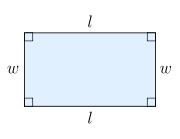The area of this rectangle is lw.

The most basic area formula is the formula for the area of a rectangle. Given a rectangle with length l and w, the formula for the area is

A = lw  (rectangle).

That is, the area of the rectangle is the length multiplied by the width. As a special case, the area of a square with side length s is given by the formula

A = s2  (square).

The formula for the area of a rectangle follows directly from the basic properties of area, and is sometimes taken as a definition or axiom. On the other hand, if geometry is developed before arithmetic, this formula can be used to define multiplication of real numbers.Equal area figures.

### Dissection formulae

Most other simple formulae for area follow from the method of dissection. This involves cutting a shape into pieces, whose areas must sum to the area of the original shape.

For an example, any parallelogram can be subdivided into a trapezoid and a right triangle, as shown in figure to the left. If the triangle is moved to the other side of the trapezoid, then the resulting figure is a rectangle. It follows that the area of the parallelogram is the same as the area of the rectangle:

A = bh  (parallelogram).Two equal triangles.

However, the same parallelogram can also be cut along a diagonal into two congruent triangles, as shown in the figure to the right. It follows that the area of each triangle is half the area of the parallelogram:$A = \frac{1}{2}bh$  (triangle).

Similar arguments can be used to find area formulae for the trapezoid and the rhombus, as well as more complicated polygons.

### Circles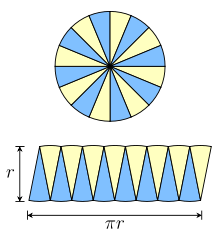A circle can be divided into sectors which rearrange to form an approximate parallelogram.

The formula for the area of a circle is based on a similar method. Given a circle of radius r, it is possible to partition the circle into sectors, as shown in the figure to the right. Each sector is approximately triangular in shape, and the sectors can be rearranged to form and approximate parallelogram. The height of this parallelogram is r, and the width is half the circumference of the circle, or πr. Thus, the total area of the circle is r × πr, or πr2:

A = πr2  (circle).

Though the dissection used in this formula is only approximate, the error becomes smaller and smaller as the circle is partitioned into more and more sectors. The limit of the areas of the approximate parallelograms is exactly πr2, which is the area of the circle.

This argument is actually a simple application of the ideas of calculus. In ancient times, the method of exhaustion was used in a similar way to find the area of the circle, and this method is now recognized as a precursor to integral calculus. Using modern methods, the area of a circle can be computed using a definite integral:$A \;=\; \int_{-r}^r 2\sqrt{r^2 - x^2}\,dx \;=\; \pi r^2.$

### Surface area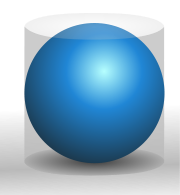Archimedes showed that the surface area and volume of a sphere is exactly 2/3 of the area and volume of the surrounding cylindrical surface.

Most basic formulae for surface area can be obtained by cutting surfaces and flattening them out. For example, if the side surface of a cylinder (or any prism) is cut lengthwise, the surface can be flattened out into a rectangle. Similarly, if a cut is made along the side of a cone, the side surface can be flattened out into a sector of a circle, and the resulting area computed.

The formula for the surface area of a sphere is more difficult: because the surface of a sphere has nonzero Gaussian curvature, it cannot be flattened out. The formula for the surface area of a sphere was first obtained by Archimedes in his work On the Sphere and Cylinder. The formula is

A = 4πr2  (sphere).

where r is the radius of the sphere. As with the formula for the area of a circle, any derivation of this formula inherently uses methods similar to calculus.

## List of formulae

Common formulae for area:
Shape Formula Variables
Regular triangle (equilateral triangle)$\tfrac14\sqrt{3}s^2\,\!$ s is the length of one side of the triangle.
Triangle$\sqrt{s(s-a)(s-b)(s-c)}\,\!$ s is half the perimeter, a, b and c are the length of each side.
Triangle$\tfrac12 a b \sin(C)\,\!$ a and b are any two sides, and C is the angle between them.
Triangle$\tfrac12bh \,\!$ b and h are the base and altitude (measured perpendicular to the base), respectively.
Square$s^2\,\!$ s is the length of one side of the square.
Rectangle$lw \,\!$ l and w are the lengths of the rectangle's sides (length and width).
Rhombus$\tfrac12ab$ a and b are the lengths of the two diagonals of the rhombus.
Parallelogram$bh\,\!$ b is the length of the base and h is the perpendicular height.
Trapezoid$\tfrac12(a+b)h \,\!$ a and b are the parallel sides and h the distance (height) between the parallels.
Regular hexagon$\tfrac32\sqrt{3}s^2\,\!$ s is the length of one side of the hexagon.
Regular octagon$2\left(1+\sqrt{2}\right)s^2\,\!$ s is the length of one side of the octagon.
Regular polygon$\frac{1}{4}nl^2\cdot \cot(\pi/n)\,\!$ l is the side length and n is the number of sides.
Regular polygon$\frac{1}{4n}p^2\cdot \cot(\pi/n)\,\!$ p is the perimeter and n is the number of sides.
Regular polygon$\frac{1}{2}nR^2\cdot \sin(2\pi/n) = nr^2 \tan(\pi/n)\,\!$ R is the radius of a circumscribed circle, r is the radius of an inscribed circle, and n is the number of sides.
Regular polygon$\tfrac12a p \,\!$ a is the apothem, or the radius of an inscribed circle in the polygon, and p is the perimeter of the polygon.
Circle$\pi r^2\ \text{or}\ \frac{\pi d^2}{4} \,\!$ r is the radius and d the diameter.
Circular sector$\tfrac12 r^2 \theta \,\!$ r and θ are the radius and angle (in radians), respectively.
Ellipse$\pi ab \,\!$ a and b are the semi-major and semi-minor axes, respectively.
Total surface area of a Cylinder$2\pi r (r + h)\,\!$ r and h are the radius and height, respectively.
Lateral surface area of a cylinder$2 \pi r h \,\!$ r and h are the radius and height, respectively.
Total surface area of a Cone$\pi r (r + l) \,\!$ r and l are the radius and slant height, respectively.
Lateral surface area of a cone$\pi r l \,\!$ r and l are the radius and slant height, respectively.
Total surface area of a Sphere$4\pi r^2\ \text{or}\ \pi d^2\,\!$ r and d are the radius and diameter, respectively.
Total surface area of an ellipsoid   See the article.
Total surface area of a Pyramid$B+\frac{P L}{2}\,\!$ B is the base area, P is the base perimeter and L is the slant height.
Square to circular area conversion$\frac{4}{\pi} A\,\!$ A is the area of the square in square units.
Circular to square area conversion$\frac{1}{4} C\pi\,\!$ C is the area of the circle in circular units.

The above calculations show how to find the area of many common shapes.

The area of irregular polygons can be calculated using the "Surveyor's formula".

### Areas of 2-dimensional figures

• a triangle:$\tfrac12Bh$ (where B is any side, and h is the distance from the line on which B lies to the other vertex of the triangle). This formula can be used if the height h is known. If the lengths of the three sides are known then Heron's formula can be used:$\sqrt{s(s-a)(s-b)(s-c)}$ where a, b, c are the sides of the triangle, and$s = \tfrac12(a + b + c)$ is half of its perimeter. If an angle and its two included sides are given, the area is$\tfrac12 a b \sin(C)$ where C is the given angle and a and b are its included sides. If the triangle is graphed on a coordinate plane, a matrix can be used and is simplified to the absolute value of$\tfrac12(x_1 y_2 + x_2 y_3 + x_3 y_1 - x_2 y_1 - x_3 y_2 - x_1 y_3)$. This formula is also known as the shoelace formula and is an easy way to solve for the area of a coordinate triangle by substituting the 3 points (x1,y1), (x2,y2), and (x3,y3). The shoelace formula can also be used to find the areas of other polygons when their vertices are known. Another approach for a coordinate triangle is to use Infinitesimal calculus to find the area.
• a simple polygon constructed on a grid of equal-distanced points (i.e., points with integer coordinates) such that all the polygon's vertices are grid points:$i + \frac{b}{2} - 1$, where i is the number of grid points inside the polygon and b is the number of boundary points. This result is known as Pick's theorem.

### Area in calculus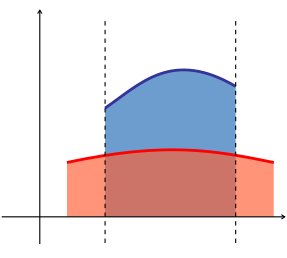The area between two graphs can be evaluated by calculating the difference between the integrals of the two functions
• the area between the graphs of two functions is equal to the integral of one function, f(x), minus the integral of the other function, g(x).
• an area bounded by a function r = r(θ) expressed in polar coordinates is${1 \over 2} \int_0^{2\pi} r^2 \, d\theta$.
• the area enclosed by a parametric curve$\vec u(t) = (x(t), y(t))$ with endpoints$\vec u(t_0) = \vec u(t_1)$ is given by the line integrals$\oint_{t_0}^{t_1} x \dot y \, dt = - \oint_{t_0}^{t_1} y \dot x \, dt = {1 \over 2} \oint_{t_0}^{t_1} (x \dot y - y \dot x) \, dt$

(see Green's theorem)

or the z-component of${1 \over 2} \oint_{t_0}^{t_1} \vec u \times \dot{\vec u} \, dt.$

### Surface area of 3-dimensional figures

• cube: 6s2, where s is the length of the top side
• rectangular box:$2 (\ell w + \ell h + w h)$ the length divided by height
• cone:$\pi r\left(r + \sqrt{r^2 + h^2}\right)$, where r is the radius of the circular base, and h is the height. That can also be rewritten as πr2 + πrl where r is the radius and l is the slant height of the cone. πr2 is the base area while πrl is the lateral surface area of the cone.
• prism: 2 × Area of Base + Perimeter of Base × Height

#### General formula

The general formula for the surface area of the graph of a continuously differentiable function z = f(x,y), where$(x,y)\in D\subset\mathbb{R}^2$ and D is a region in the xy-plane with the smooth boundary:$A=\iint_D\sqrt{\left(\frac{\partial f}{\partial x}\right)^2+\left(\frac{\partial f}{\partial y}\right)^2+1}\,dx\,dy.$

Even more general formula for the area of the graph of a parametric surface in the vector form$\mathbf{r}=\mathbf{r}(u,v),$ where$\mathbf{r}$ is a continuously differentiable vector function of$(u,v)\in D\subset\mathbb{R}^2$:$A=\iint_D \left|\frac{\partial\mathbf{r}}{\partial u}\times\frac{\partial\mathbf{r}}{\partial v}\right|\,du\,dv.$

## Minimization

Given a wire contour, the surface of least area spanning ("filling") it is a minimal surface. Familiar examples include soap bubbles.

The question of the filling area of the Riemannian circle remains open.

Wikimedia Foundation. 2010.

Synonyms:

### Look at other dictionaries:

• area — ar‧e‧a [ˈeəriə ǁ ˈeriə] noun [countable] 1. a part of a city or country: • What area of Bristol do you live in? • the New York metropolitan area (= New York city and the area around it ) asˌsisted ˈarea …   Financial and business terms

• Área 69 — Area 69 promocionando 12 RAZONES PARA… Datos generales …   Wikipedia Español

• área — sustantivo femenino 1. Espacio de tierra entre ciertos límites: Un área extensa del campo se dedica al cultivo de hortalizas. 2. Espacio o zona donde se dan determinados fenómenos o características: área urbana, área de bajas presiones, área medi …   Diccionario Salamanca de la Lengua Española

• Área 7 — Datos generales Origen Lima, Perú Estado En actividad …   Wikipedia Español

• área — s. f. 1. Extensão compreendida dentro de certos limites. 2. Vão que sobe de um saguão interior até ao telhado da casa. 3. Espaço (em que se exerce determinada ação). 4. Halo. 5. Espaço que percorre em certo tempo o raio vetor de um astro. 6. … …   Dicionário da Língua Portuguesa

• Area 52 — may refer to: * Tonopah Test Range a military installation located about 30 miles southeast of Tonopah, Nevada. * Stargate Command, canon codename Area 52, located under NORAD * The Top Secret United States government facility similar to Area 51… …   Wikipedia

• AREA — (лат.), поле, участок, место, в неврологии этим термином обознач. нек рые участки центо. Нервной системы (см. ниже). Area acustica, область, расположенная по дну IV желудочка, в наружном его углу, на границе Ва ролиева моста и продолговатого… …   Большая медицинская энциклопедия

• area — 1 Area, tract, region, zone, belt mean an extent of space especially of ground or surface that is distinguishable from its surroundings in appearance or in certain distinctive features. Area still carries its original implication of clearly… …   New Dictionary of Synonyms

• area — ⇒AREA, subst. fém. A. ARCHIT. [Dans les monastères de l Église d Orient] Surface libre entourant l église et que délimite le cloître : • 1. Le cloître des monastères de l Orient est l enceinte qui enveloppe l area ou surface libre, dont l église… …   Encyclopédie Universelle

• Area — A re*a ([=a] r[ e]*[.a]; 277), n.; pl. {Areas} ( [.a]z) . [L. area a broad piece of level ground. Cf. {Are}, n.] 1. Any plane surface, as of the floor of a room or church, or of the ground within an inclosure; an open space in a building. [1913… …   The Collaborative International Dictionary of English

• Area 19 — is one of a number of nuclear test areas within the Pahute Mesa section of the Nevada Test Site (NTS). Pahute Mesa is one of four major nuclear test regions within the (NTS) and it encompasses Area 19 and Area 20.Both Area 19 and Area 20 were… …   Wikipedia